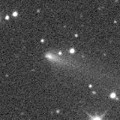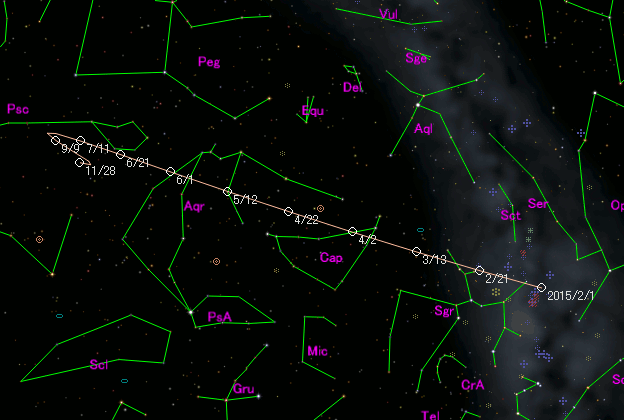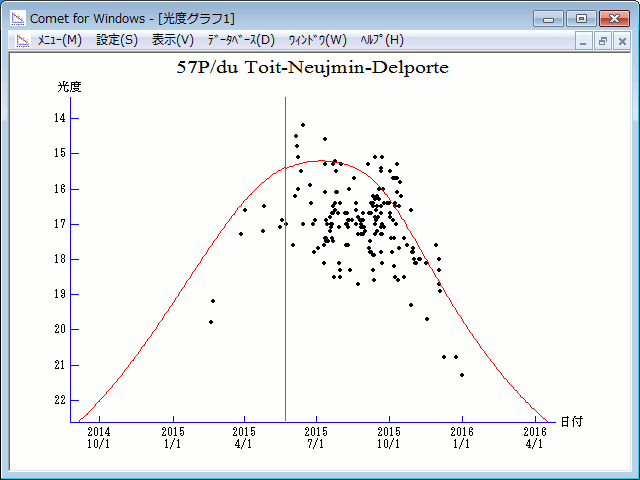# \$B%I%%!&%H%\$%H(B-\$B%K%'%&%\$%_%s(B-\$B%G%k%]%k%HWB@1(B

57P/du Toit-Neujmin-Delporte (2015)###\$B###\$B50F;MWAG(B

```Epoch 2015 May 18.0 TT = JDT 2457160.5
T 2015 May 22.28387 TT                                  MPCW
q   1.7288551            (2000.0)            P               Q
n   0.15362583     Peri.  115.22595     +0.55941274     +0.82885438
a   3.4527084      Node   188.80106     -0.77725189     +0.52135425
e   0.4992757      Incl.    2.84809     -0.28798764     +0.20295359
P   6.42
From 959 observations 1996 July 24-2015 Dec. 31, mean residual 0".7.
Nongravitational parameters A1 = +0.50, A2 = -0.0071.
```

###\$B@1?^(B###\$B8wEYJQ2=(B

```        m1 = 8.0 + 5 log\$B&\$(B + 27.0 log r  [ ,0]  (              \$B!A(B2015\$BG/(B 5\$B7n(B22\$BF|(B)
m1 = 9.7 + 5 log\$B&\$(B + 20.0 log r  [0, ]  (2015\$BG/(B 5\$B7n(B22\$BF|!A(B              )
```##### \$B50F;MWAG\$O(BM.P.E.C. 2016-D32\$B\$K7G:\\$5\$l\$?\$b\$N\$G\$9!#(B \$B@1?^\$O%9%F%i%J%S%2!<%?(B Ver.8 (\$B%"%9%H%m%"!<%D(B) \$B\$G:n@.\$7\$?\$b\$N\$G\$9!#(B \$B8wEY%0%i%U\$O(BComet for Windows\$B\$G:n@.\$7\$?\$b\$N\$G\$9!#(B